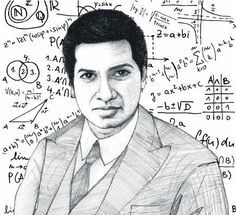# My math notes - Part 1

## Sequence

https://en.wikipedia.org/wiki/Sequence

• Sequence is like list
• Often write like this: $a_n, b_n, c_n$

Example: $\{(p_t, v_t)_{t=1,...,T}\}$ where $p_t$ is the price associated with tick t and $v_t$ is the volume associated with tick t. The so-called tick rule defines a sequence $\{b_t\}_{t=1,...,T}$ where

$b_t= \begin{cases} b_{t-1} &\text{if $$\Delta{p_t}=0$$} \\ \frac{\Delta{p_t}}{\Delta{p_t}} &\text{if $$\Delta{p_t}\neq0$$} \end{cases}$

# Đứa con của Thượng đế - Ramanujan## I. Thuở sơ khai

Ngày xưa mình đọc được trong những quyển sách, bài báo, rằng có những người đạt được những năng lực tưởng như siêu nhiên, kiểu như thần đồng toán học không cần ai chỉ dạy cũng có thể hiểu và làm được những phép toán kỳ diệu.

Mình thì mình không tin đâu.

# Pi

Số pi1 (ký hiệu: π) là một hằng số toán học có giá trị bằng tỷ số giữa chu vi của một đường tròn với đường kính của đường tròn đó. Hằng số này có giá trị xấp xỉ bằng 3,14159265358979. Nó được biểu diễn bằng chữ cái Hy Lạp π từ giữa thế kỉ 18.
π là một số vô tỉ, nghĩa là nó không thể được biểu diễn chính xác dưới dạng tỉ số của hai số nguyên. Nói cách khác, nó là một số thập phân vô hạn không tuần hoàn. Hơn nữa, π còn là một số siêu việt - tức là nó không phải là nghiệm của bất kì đa thức với hệ số hữu tỉ nào. Tính siêu việt của π kéo theo sự vô nghiệm của bài toán cầu phương. Các con số trong biểu diễn thập phân của π dường như …